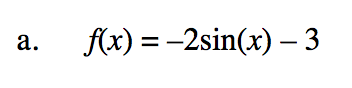### Home > PC3 > Chapter 8 > Lesson 8.3.1 > Problem8-90

8-90.
1. Graph two complete cycles of each of the following trigonometric functions. Then state the amplitude, period, and any shifts or reflections of the graph. Homework Help ✎

1. f(x) = –2sin(x) – 3

2. f(x) = 5cos(π(x – 5))For each function, identify the amplitude, period, horizontal shift, vertical shift, and any reflection.
Then sketch the function. Check your sketch using a graphing calculator. Identify and correct any mistakes.